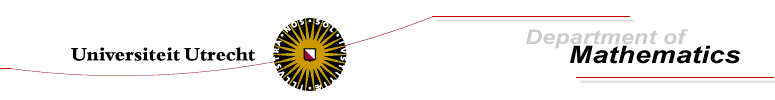Errata for Contact Structures of Partial Differential Equations

p. 8:
Remark 1.2.15: line 6: dot should be a comma
p. 39:
Section 2.3: line 2: \Gr_k instead of \Gr_2
p. 49:
line -7: x_0 should be L_0
p. 52:
line 1: 'compact hyperbolic surface' should be 'connected hyperbolic surface'
Example 2.3.7: line 2: remove "compact"
p. 53:
Example 2.3.8: "over" should be "cover"
p. 58:
line -6: l_2(b_0) should be l_1(b_0)
p. 59:
Example: Compact surfaces of type (2', 3'):
• Roughly 9th line: \pi_2 should be \pi_{1,3}
• The dot after the definition of F'_L should be removed
• line -10: \pi_2 \comp \sigma should be \pi_2' \comp \sigma
• line -7: 'This is map' should be ' This is a map'
p. 62:
q_{r1} should be r_{11}
p. 63-65
The term 'free' should be 'effective' (occurs multiple times)
p. 63:
fourth display on page: indices should be subscript
p. 63:
line -6: q_{22} should be r_{22}
p. 65:
line 3: two out of should read one out of
p. 70:
In formula 2.22, structure equation for \eta'': \eta' \wedge \eta'' should be eta' \wedge \zeta''.
p. 141
In the section on hyperbolic exterior differential systems we do not require the hyperbolic exterior differential systems to be closed. In the literature it is more common to require an hyperbolic EDS to be closed.
p. 192:
comma in first display